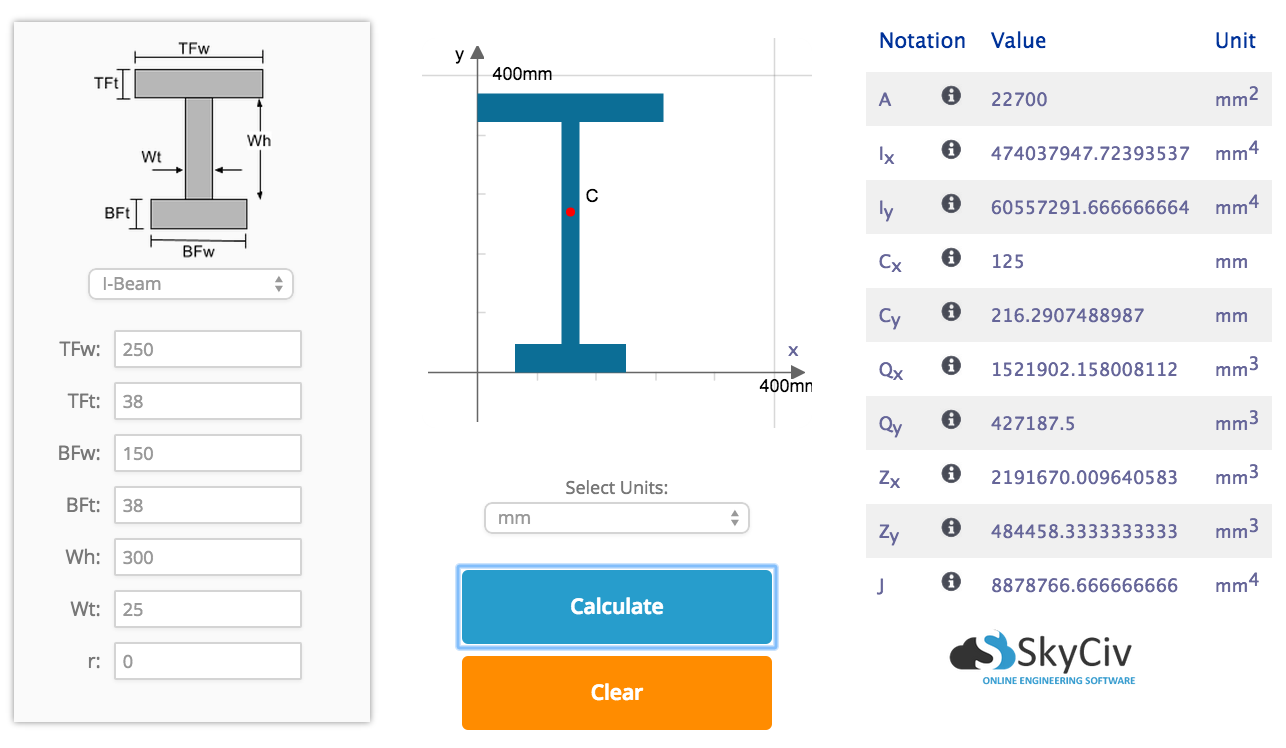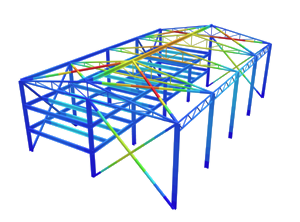SkyCiv Documentation

Your guide to SkyCiv software - tutorials, how-to guides and technical articles

1. Home
2. Tutorials
3. Section Tutorials
4. Calculating the Moment of Inertia of a Beam Section

# Calculating the Moment of Inertia of a Beam Section

## How to Calculate the Moment of Inertia of a Beam Section(Second Moment of Area)

Before we find the moment of inertia (or second moment of area) of a beam section, its centroid (or center of mass) must be known. For instance, if the moment of inertia of the section about its horizontal (XX) axis was required then the vertical (y) centroid would be needed first (Please view our Tutorial on how to calculate the Centroid of a Beam Section).

Before we start, if you were looking for our Free Moment of Inertia Calculator please click the link to learn more. This will calculate the centroid, moi and other results and even show you the step by step calculations! But for now, let’s look at a step-by-step guide and example of how to calculate moment of inertia:

## Step 1: Segment the beam section into parts

When calculating the area moment of inertia, we must calculate the moment of inertia of smaller segments. Try to break them into simple rectangular sections. For instance, consider the I-beam section below, which was also featured in our Centroid Tutorial. We have chosen to split this section into 3 rectangular segments: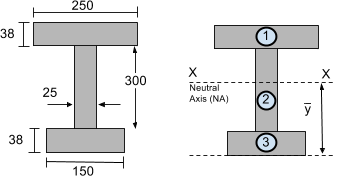## Step 2: Calculate the Neutral Axis (NA)

The Neutral Axis (NA) or the horizontal XX axis is located at the centroid or center of mass. In our Centroid Tutorial, the centroid of this section was previously found to be 216.29 mm from the bottom of the section.

## Step 3: Calculate Moment of Inertia

To calculate the total moment of inertia of the section we need to use the “Parallel Axis Theorem”: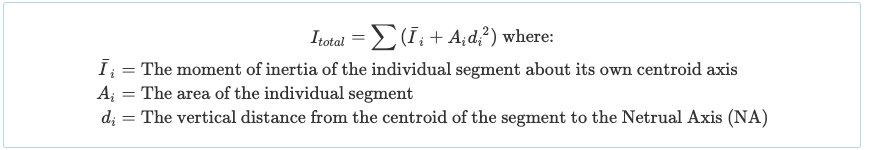Since we have split it into three rectangular parts, we must calculate the moment of inertia of each of these sections. It is widely known that the moment of inertia equation of a rectangle about its centroid axis is simply:The moment of inertia of other shapes are often stated in the front/back of textbooks or from this guide of moment of inertia shapes. However the rectangular shape is very common for beam sections, so it is probably worth memorizing.

Now we have all the information we need to use the “Parallel Axis Theorem” and find the total moment of inertia of the I-beam section. In our moment of inertia example: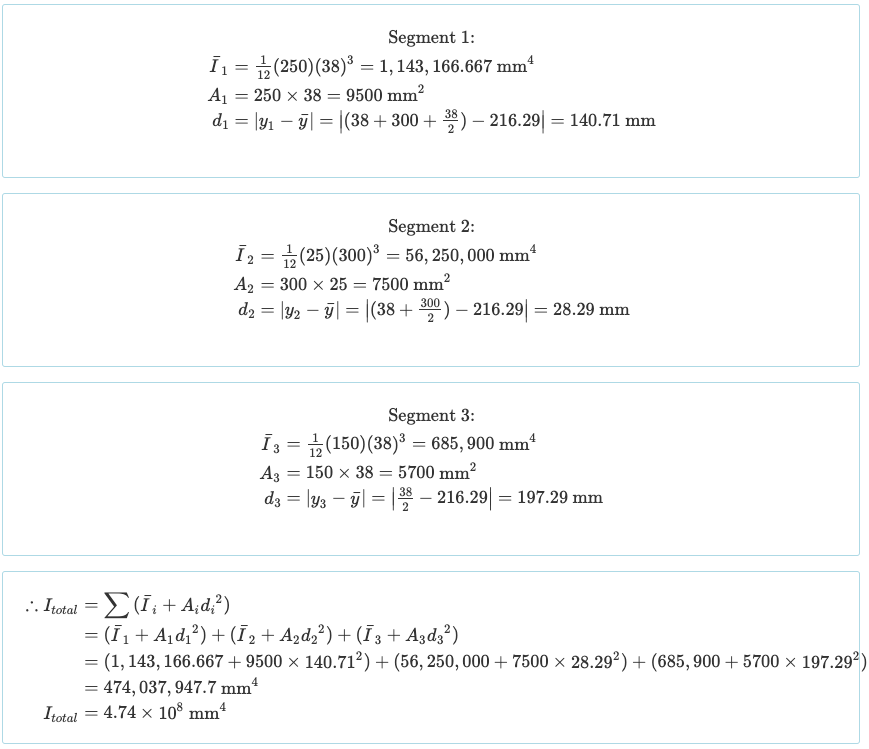So there you have our guide on calculating the area of moment for beam sections. This result is critical in structural engineering and is an important factor in the deflection of a beam. We hope you enjoyed the tutorial and look forward to any comments you have.

## BONUS: Using our Moment of Inertia Calculator

SkyCiv’s Account shows full calculations of moment of inertia. This interactive module will show you the step-by-step calculations of how to find moment of inertia: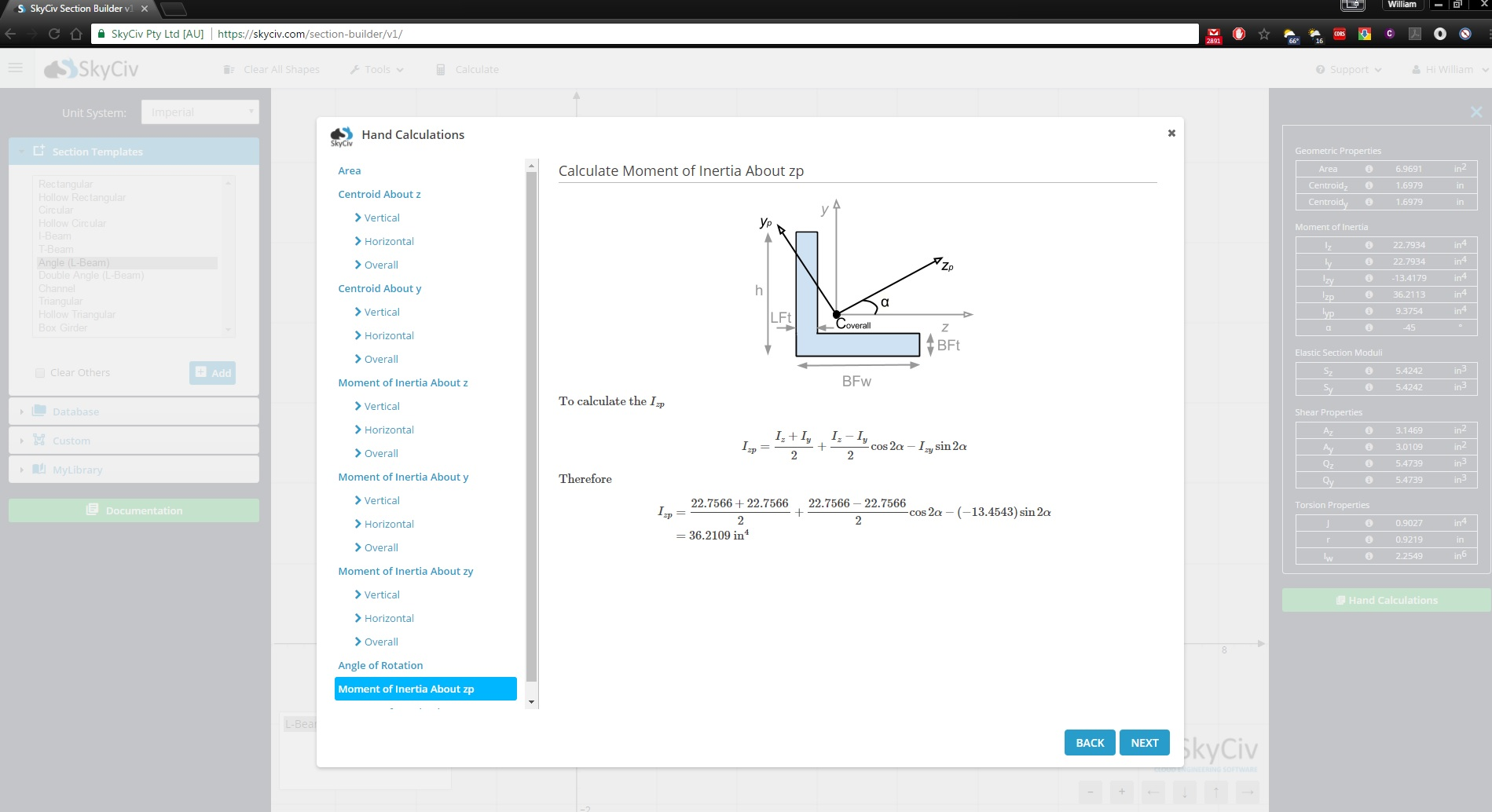Alternatively, you can see the results of our Free Moment of Inertia Calculator to check your work. This will calculate all the properties of your cross section and is a useful reference to calculate the Centroid, Area and Moment of Inertia of your beam sections!